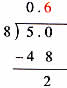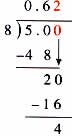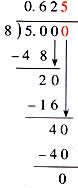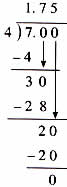Thursday , July 7 2022# NCERT 5th Class (CBSE) Mathematics: Decimals

### D. Dividing a whole Number by a whole Number with a Decimal Quotient

(a) Rita’s mother backed a cake and cut it into 8 pieces. Rita took 5 pieces to share with her friends in school. How would you express the amount Rita took to school as a fraction and then as a decimal?

5/8 as a fraction

5÷8 = ?

8 ) 5 (               8 ) 5.0 ( .Answer: Rita took 5/8 or 0.625 of the cake to school.

(b) 7÷4 = ?### Dividing by Decimal Numbers

Look at this division of a whole number.        7 ) 28 ( 4

Multiply both the dividend by 10.                        70 ) 280 (4

Multiply both the divisor and the dividend by 100.          700 ) 2800 (4

The quotient has changed.

#### When you divide by decimals, you need to first change the divisor into a whole number.

(a) 0.76÷04 = ?

Step 1. Multiply the decimal divisor by a multiple of 10 to make it a whole number.        0.4×10 = 4

Step 2. Multiply the dividend by the same multiple of 10.                 0.76×10 =7.6

Image:

Step 3. Divide as usual.